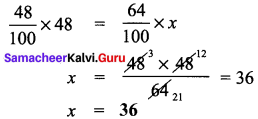# Samacheer Kalvi 8th Maths Solutions Term 2 Chapter 1 Life Mathematics Ex 1.1

Students can Download Maths Chapter 1 Life Mathematics Ex 1.1 Questions and Answers, Notes Pdf, Samacheer Kalvi 8th Maths Book Solutions Guide Pdf helps you to revise the complete Tamilnadu State Board New Syllabus and score more marks in your examinations.## Tamilnadu Samacheer Kalvi 8th Maths Solutions Term 1 Chapter 1 Life Mathematics Ex 1.1

Question 1.
Fill in the blanks:

Question (i)
If 30% of x is 150, then x is ………….
500
Hint:
Given 30% of x is 150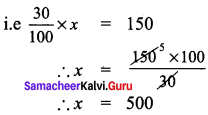Question (ii)
2 minutes is ………… % to an hour.
3$$\frac{1}{3}$$%
Hint:
Let 2 min be x% of an hour
and 1 hr = 60 min
x% = $$\frac{2}{60}$$ x 100 = $$\frac{200}{3}$$ = $$\frac{10}{3}$$ = 3$$\frac{1}{3}$$
x = 3$$\frac{1}{3}$$Question (iii)
If x % of x = 25, then x = …………
50
Hint:
Given that x % of x is 25
∴ $$\frac{x}{100}$$ = 25
x2 = 25 x 100 = 2500
∴ x = $$\sqrt{2500}$$ = 50

Question (iv)
In a school of 1400 students, there are 420 girls. The percentage of boys in the school is ………….
70
Hint:
Given total number of students in school = 1400
Number of girls in school = 420
Number of boys in school = 1400 – 420 = 980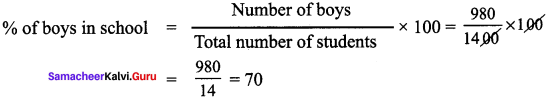% of boys = 70%

Question (v)
0.5252 is ………….. %.
52.52%
Hint:
Given a number, and to express as a percentage, we need to multiply by 100
∴ to express 0.5252 as percentage, we should multiply by 100
∴ 0.5252 x 100 = 52.52%

Question 2.
Rewrite each underlined portion using percentage language.

Question (i)
One half of the cake is distributed to the children.
50% of the cake is distributed to the children
Hint:
One half is nothing but $$\frac{1}{2}$$ as percentage, we need to multiply by 100
$$\frac{1}{2}$$ x 100 = 50%Question (ii)
Aparna scored 7.5 points out of 10 in a competition.
Aparna scored 75% in a competition
Hint:
7.5 points out of 10 is $$\frac{7.5}{10}$$ = 0.75
For percentage, we need to multiply by 100
∴ We get 0.75 x 100 = 75%

Question (iii)
The statue was made of pure silver.
96% students participated in sports
Hint:
Pure silver means there are no other metals
so, 100 out of 100 parts is made of silver = $$\frac{100}{100}$$
∴ to express as percentage, $$\frac{100}{100}$$ x 100% = 100%

Question (iv)
48 out of 50 students participated in sports
96% students participated in sports
Hint:
48 out of 50 students in fraction form is $$\frac{48}{50}$$.
As a percentage, we need to multiply by 100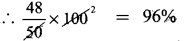Question (v)
Only 2 persons out of 3 will be selected in the interview
Only 66$$\frac{2}{3}$$% persons will be selected in the interview.
Hint:
2 out of 3 in fraction form is $$\frac{2}{3}$$ to express as percentage, we need to multiply by 100
$$\frac{2}{3}$$ x 100 = $$\frac{200}{3}$$ = 66$$\frac{2}{3}$$%

Question 3.
48 is 32% of what number?
Solution:
Let the number required to be found be ‘x’
Given that 32% of x is 48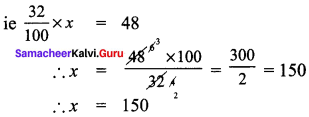Question 4.
A bank pays ₹ 240 as interest for 2 years for a sum of ? 3000 deposited as savings. Find the rate of interest given by the bank.
Solution:
The formula for simple interest is given by
Interest (I) = $$\frac{PxRxT}{100}$$ 100
Where P is principal amount; R is rate of interest in percentage; t is time period Substituting given values in formula, we get
P = 3000, t = 2 yrs, I = Rs 240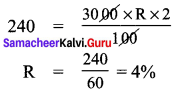Question 5.
A Welfare Association has a sports club where 30% of the members play cricket, 28% play volleyball, 22% play badminton and the rest play indoor games. If 30 member play indoor games.

1. How many members are there in the sports club?
2. How many play cricket, volleyball and badminton?

Solution:
Given data
Members who play Cricket = 30% …..(1)
Members who play Vollyball = 28% …..(2)
Members who play Batminton = 22% ……(3)
Members who play outdoor games = (1) + (2) + (3) = 30
Members who play indoor games = 100 – 80 = 20%
Also given 30 members play indoor games
Let total no of members be ‘x’
∴ $$\frac{30}{x}$$ = 20
∴ x = $$\frac{30×100}{20}$$ = 15

1. Total number of members is 150

2. Members who play Cricket = 30% of total = $$\frac{30}{100}$$ x 150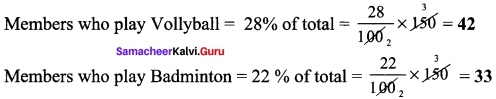Question 6.
What is 25% of 30% of 400?
Solution:
Required to find 25% of 30% of 400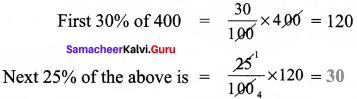Question 7.
If the difference between 75% of a number and 60% of the same number is 82.5, then find 20% of the number.
Solution:
Given that 75% of number less 60% of number is 82.5. Let the number be ‘x’
∴ $$\frac{75}{100}$$ × x = 82.5
∴ 0.75 – 0.6.60x = 82.5
∴ 0.15x = 82.5
∴ x = $$\frac{82.5}{0.15}$$ = $$\frac{8250}{15}$$ = 550
Required to find 20% of number ie 20% of x.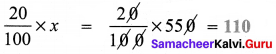Question 8.
If a car is sold for ₹ 2,00,000 from its original price of? 3,00,000 find the percentage for decrease in the value of the car.Solution:
Original price of car = ₹ 3,00,000
actual selling price of car = ₹ 2,00,000
Decrease in amount from original = 3,00,000 – 2,00,000 = 1,00,000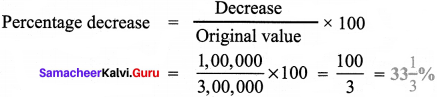Question 9.
A number when increased by 18% gives 236. Find the number.
Solution:
Let the number be x. Given that when it is increased by 18%, we get 236.
x + $$\frac{18}{100}$$ = 236
$$\frac{100x+18x}{100}$$ = 236
$$\frac{118}{100}$$x = 236
The number = x = $$\frac{236×100}{118}$$ = 200Question 10.
A number when decreased by 20% gives 80. Find the number.
Solution:
Let the number be x. Given that when it is increased by 20% we get 80.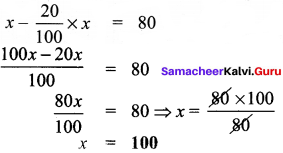Question 11.
A number is increased by 25% and then decreased by 20%. Find the change in that number.
Solution:
Method 1:
Let the number be x. First it is increased by 25%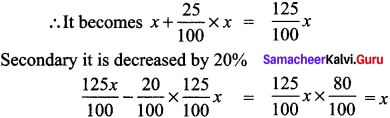Method 2:
[to understand, let us assume that number is 100]
So, first when we increase by 25%, we get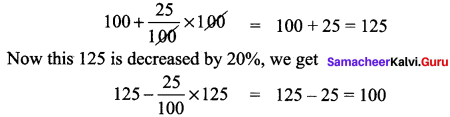We get back 100 ⇒ No change

Question 12.
If the numerator of a fraction is increased by 25% and the denominator is increased by 10%, it becomes $$\frac{2}{5}$$. Find the original fraction.
Solution:
Let the fraction be $$\frac{N}{D}$$ where N is numerator & D is denominator
It is given that numerator is include by 25%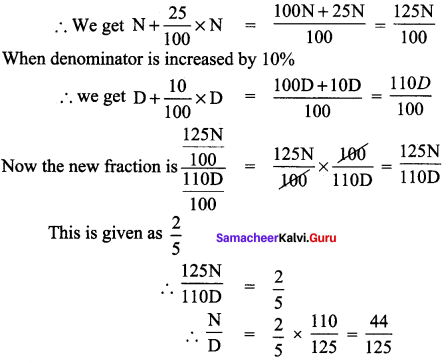Question 13.
A fruit vendor bought some mangoes of which 10% were rotten. He sold 33 $$\frac{1}{3}$$% of the rest. Find the total number of mangoes bought by him initially, if he still has 240 mangoes with him.Solution:
Let the number of mangoes bought by fruit seller initially be x.
Given that 10% of mangoes were rotten
∴ Number of rotten mangoes = $$\frac{10}{100}$$ × x
Number of good mangoes = x – no. of rotten mangoes
= x – $$\frac{10}{100}$$x = $$\frac{100x-10x}{100}$$ = $$\frac{90}{100}$$x …(1)
Number of mangoes sold = 33$$\frac{1}{3}$$% of good mangoes = $$\frac{100}{3}$$%
∴ Mangoes sold = $$\frac{100}{3}$$ x $$\frac{90}{100}$$x × $$\frac{1}{100}$$ = $$\frac{30}{100}$$x
Number of mangoes remaining = No. of good mangoes – No. of mangoes sold
From (1) and (2)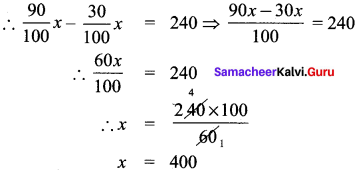Question 14.
A student gets 31% marks in an examination but fails by 12 marks. If the pass percentage is 35%, find the maximum marks of the examination.
Solution:
Let the maximum marks in the exam be ‘x’. Pass percentage is given as 35%
∴ Pass mark = $$\frac{35}{100}$$ × x = $$\frac{35}{100}$$x
Student gets 31 % marks = $$\frac{31}{100}$$ × x = $$\frac{31}{100}$$x
But student fails by 12 marks → meaning his mark is 12 less than pass mark.
∴ $$\frac{31}{100}$$x = $$\frac{35}{100}$$x – 12
∴ $$\frac{35}{100}$$x – $$\frac{31}{100}$$x = 12
∴ $$\frac{35x-31x}{100}$$ = 12 ⇒ $$\frac{4x}{100}$$ = 12
∴ $$\frac{12×100}{4}$$ = 300Question 15.
The ratio of boys and girls in a class is 5:3. If 16% of boys and 8% of girls failed in an examination, then find the percentage of passed students.
Solution:
Let number of boys be ‘6’ and number of girls be ‘g’
Ratio of boys and girls is given as 5:3
b:g = 5:3 ⇒ $$\frac{b}{g}$$ = $$\frac{5}{3}$$ …..(A)
Failure in boys = 16% = $$\frac{16}{100}$$ x b = $$\frac{16b}{100}$$
Failure in girls = 8% = $$\frac{8}{100}$$ x g = $$\frac{8g}{100}$$
Pass in boys = 100 – 16% = 84% $$\frac{84}{100}$$b …..(1)
Pass in girls = 100 – 8% = 92% = $$\frac{92}{100}$$g
From A, we have $$\frac{b}{g}$$ = $$\frac{5}{3}$$, = adding 1 on both sides, we get
$$\frac{b}{g}$$ + 1 = $$\frac{5}{3}$$ + 1
$$\frac{b+g}{g}$$ = $$\frac{5+3}{3}$$ = $$\frac{8}{3}$$
∴ g = $$\frac{3}{8}$$(b + g)
Similarly b = $$\frac{5}{8}$$(b + g)
Total pass = Pass in girls + Pass in boys
= (1) + (2) = $$\frac{84}{100}$$b + $$\frac{92}{100}$$g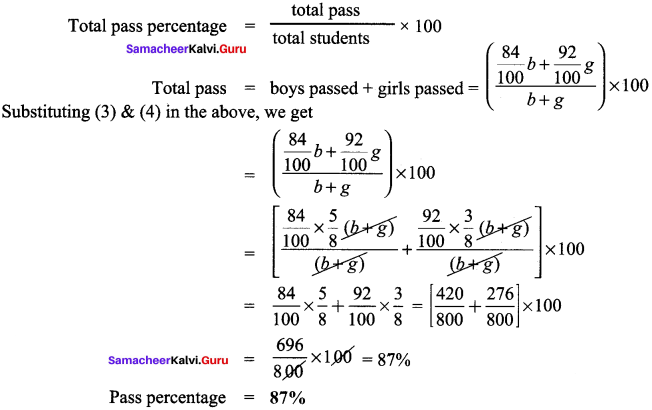Objective Type Questions

Question 16.
12% of 250 litres is the same as ………. of 150 liters
(a) 10%
(b) 15%
(c) 20%
(d) 30%
(c) 20%
Hint:
12% of 250 = $$\frac{12}{100}$$ x 250 = 30 lit
Percentage : $$\frac{30}{150}$$ x 100 = 20%

Question 17.
If three candidates A, B and C is 3 in a school election got 153, 245 and 102 votes respectively, the percentage of votes for the winner is ……..
(a) 48%
(b) 49%
(c) 50%
(d) 45%
(b) 49%
Hint:
Candidate 1: 153
Candidate 2: 245 – winner [as maximum votes]
Candidate 3: 102
Total votes = 1 + 2 + 3 = 153 + 245 + 102 = 500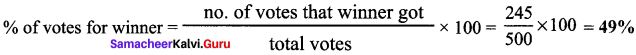Question 18.
15% of 25% of 10000 = ………..
(a) 375
(b) 400
(c) 425
(d) 475
(a) 375
Hint:
15% of 25% of 10000 is
First let us do 25% of 10,000, which is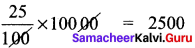Next 15% of the above is $$\frac{15}{100}$$ x 2500 = 375

Question 19.
When 60 is subtracted from 60% of a number to give 60, the number is –
(a) 60
(b) 100
(c) 150
(d) 200
(d) 200
Hint:
Let the number be ‘x’.
60% of the number is $$\frac{60}{100}$$ × x = $$\frac{60x}{100}$$
Given that when 60 is subtracted from 60%, we get 60
i.e $$\frac{60}{100}$$x – 60 = 60
∴ $$\frac{60}{100}$$x = 60 + 60 = 120
∴ x = $$\frac{120×100}{60}$$ = 200Question 20.
If 48% of 48 = 64% of x, then x =
(a) 64
(b) 56
(c) 42
(d) 36